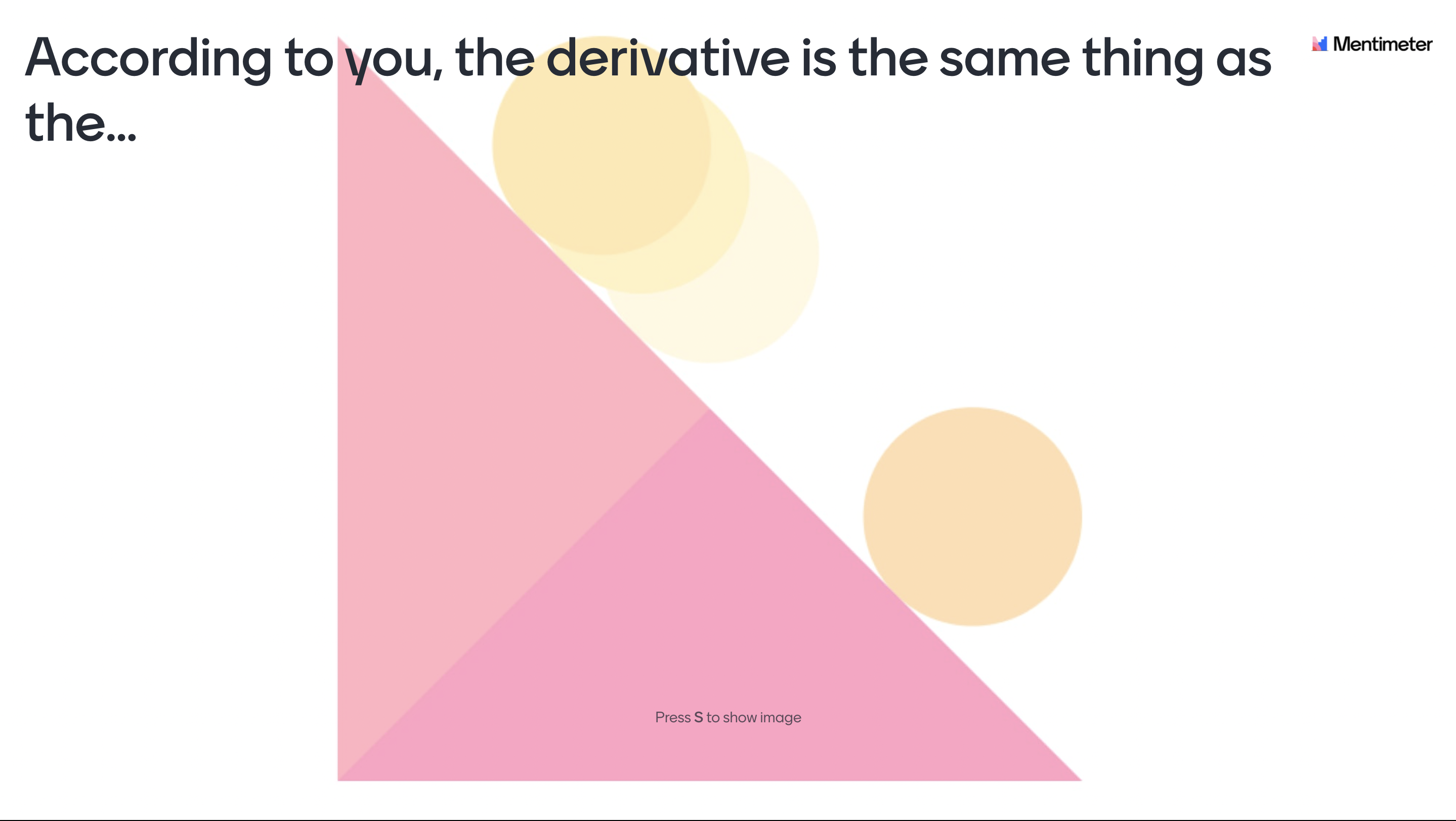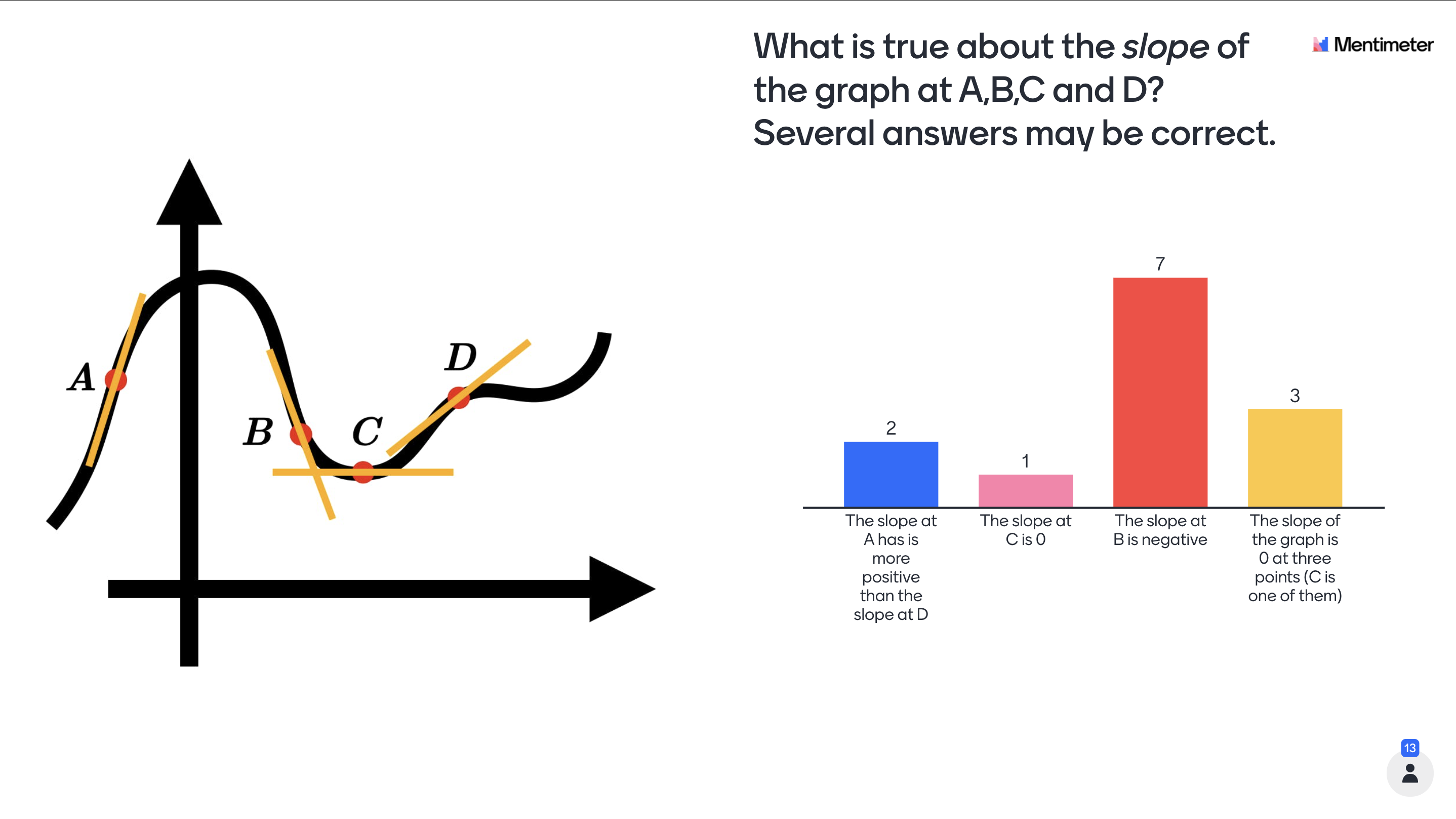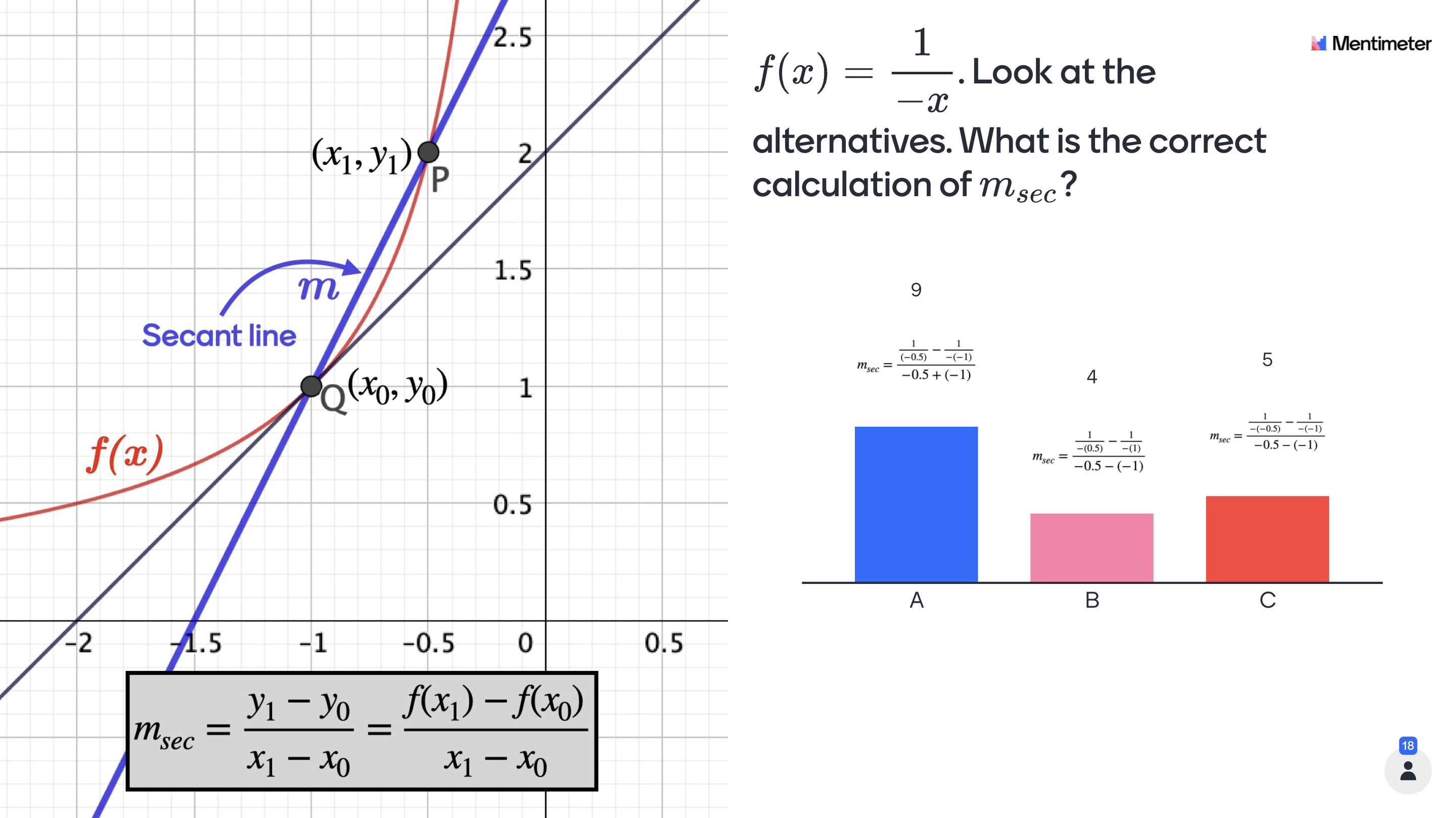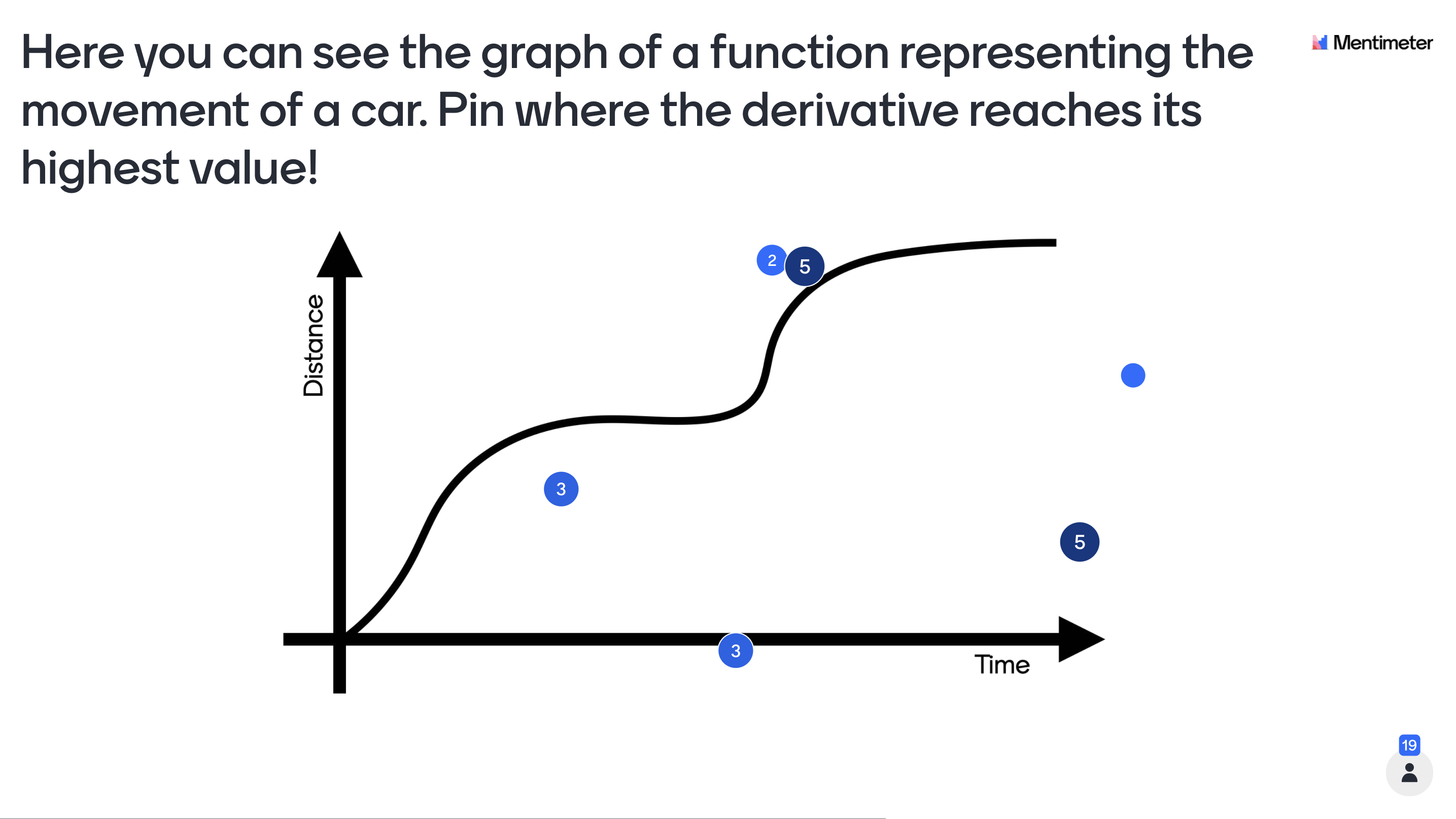# The concept of derivatives

This Mentimeter template elaborates with the concept of derivatives and the intended target group is students in 11th grade (US Curriculum). The template is designed foremost as an introduction but can also be used in the middle or as a form of reinforcement at the end of a subject block surrounding derivatives.## How to use the template

Here follows some ideas on how the slides are intended to be used but feel free to use the template however you like. Included in each cell is the purpose and other information that could be of use for you as a teacher. A general idea regarding all questions is that the students should work after the model Think-Pair-Share (TPS). When implementing TPS the students should first answer the question individually, then discuss in pairs (or groups of three) followed by a classroom discussion led by the teacher. As a concluding remark, the content in the slides are designed primarily for developing students' understanding of mathematical concepts rather than the procedural 'know how'. Therefore, discussions and questions from the students is a central part of using the presentation where your expertise as a teacher is important to clarify misconceptions and lead the classroom discussions.## Theoretical background

The concept of a derivative is an essential part of calculus, and has enormous practical applications. However, research has shown that it is very common among students to not fully grasp the concept. It is theorized that this is due in part because of a lack of prerequisite knowledge. Asiala et al. (1997) have investigated students’ conceptions of derivatives. They stress the importance that students have a rich conception of a function, including the f(x)-notation. Other things they mention as important prerequisites are the graphical representation of a slope of a line in a graph, and points in a coordinate system. Therefore, the concept of a slope gets a lot of attention in the early parts of the template in order to fill potential gaps of knowledge. Also Asiala et al. (1997) state it is important for students to see: “the relationship between the derivative of a function at a point and the slope of the line tangent to the graph of the function at that point”. This visual representation is also a core part of the presentation.Slide 1 - Introduction: Introduce the theme of the lecture and present the learning goals. For example you could mention that derivatives is an essential part of calculus that have many practical implications.

Slide 2 - General information: Inform the students regarding the TPS methodology, that their responses are anonymous and how they can access the interactive presentation.

Slide 3 - Criteria for success: Introduce a ‘criteria for success’ for the lesson with a problem that will be revisited and solved at the end of the lesson. The idea here is to make the learning visible, so the students can see for themselves that they’ve actually learned something.

Slide 4 - According to the students, a derivative is...: Let the students individually write down how they see the concept of a derivative. This can help clarify potential misconceptions.Slide 5 - Prerequisites for this lesson. [Content slide]: Repetition of the concept of a slope. Research has shown that this is an important prerequisite to be able to understand derivatives.

Slide 6 - Understanding slope: This slide presents an opportunity for thinking about what a slope really means in mathematical terms, and you as a teacher get a chance to see if your students actually feel comfortable calculating the slope.

Slide 7 - The slope of points in nonlinear functions: Introduce what a tangent line is. This is a key concept in order to get a visual understanding of the derivative.

Slide 8 - The slope at points in nonlinear functions: This slide reinforces the concept of tangent lines, and how a tangent line can be used to think about the slope at a point in a nonlinear function.Slide 9 - The slope of a secant line: Introduce the concept of a secant line. This is a key concept for understanding the derivative.

Slide 10 - Calculating the slope of the secant line using only the x-value and the function: This is a key slide! Here the students need to understand how the secant line can be calculated using only x0 and x1, and the function (without direct information of y0 and y1). It is very important that all students grasp the content of this slide in order to be able to grasp the limit definition of the derivative.

Slide 11 - Decreasing the distance between the points of the secant line: This is a key slide! The idea here is to make the students think about what will happen when the distance between the two points on the secant line becomes really small.

Slide 12 - The secant line and tangent line becomes the same [Content slide]: Give a visual representation of what happens when the distance between the two points on the secant line becomes really small.Slide 13 - Going from the secant line to the tangent line. [Content slide]: Go from the definition of the secant line to an expression for the tangent line. This is key for understanding the derivative as the slope of the tangent line at a point in a graph.

Slide 14 - Introduce the limit definition of a derivative [Content slide]: This is a key slide! Go from the specific case with two points (Q and P) to the general case. Talk about the concept of a limit. Here it can be a good idea to let the students write down the limit definition of the derivative.

Slide 15 - Derivative in a practical situation: Here the students will need to use their graphical understanding of the derivative as the slope of the tangent line at different points along the graph. Also, they will start to think about practical implications of the derivative.

Slide 16 - Using the limit definition: This is a key slide! Here you as a teacher can get a sense of the current level of understanding. Be sure to spend a lot of time giving clarifications what the expression for the derivative (2x) really means. This is essential information for the next slide.Slide 17 - Criteria for success revisited: Return to the problem that was introduced in the beginning of the lecture to make the students realize they’ve actually learnt something.

Slide 18 - Q&A: Let the students individually write down what they still feel is unclear about derivatives.

Slide 16-24 - Quiz competition! Instruct the students to enter their real names. Let the students compete in a fun way while you as a teacher can get a sense of the individual level of knowledge. This information could be used to give support for students in need of extra guidance.

## Explore more templates### The concept of functions

Pro### The concept of logarithms

ProPro